NCERT Solutions for Class 10 Science Chapter 11 Human Eye And Colorful WorldWritten by Team Trustudies
Updated at 2021-05-07

NCERT solutions for class 10 Science Chapter 11 Human Eye And Colorful World Exercise

Q1 )

The human eye can focus objects at different distances by adjusting the focal length of the eye lens. This is due to

1. presbyopia
2. accommodation
3. near-sightedness
4. far-sightedness

NCERT Solutions for Class 10 Science Chapter 11 Human Eye And Colorful World

(b)accommodation

Q2 )

The human eye forms the image of an object at its

1. cornea
2. iris
3. pupil
4. retina

NCERT Solutions for Class 10 Science Chapter 11 Human Eye And Colorful World

(d)retina

Q3 )

The least distance of distinct vision for a young adult with normal vision is about

1. 25m
2. 2.5cm
3. 25cm
4. 2.5m

NCERT Solutions for Class 10 Science Chapter 11 Human Eye And Colorful World

(c)25cm

Q4 )

The change in focal length of an eye lens is caused by the action of the

1. pupil
2. retina
3. ciliary muscles
4. iris

NCERT Solutions for Class 10 Science Chapter 11 Human Eye And Colorful World

(c)ciliary muscles

Q5 ) A person needs a lens of power -5.5 dioptres for correcting his distant vision. For correcting his near vision he needs a lens of power +1.5 dioptre. What is the focal length of the lens required for correcting (i) distant vision, and (ii) near vision ?

NCERT Solutions for Class 10 Science Chapter 11 Human Eye And Colorful World

The power P of a lens of focal length f is given by the relationP= $\frac{1}{f}$

(i) Power of the lens used for correcting distant vision = - 5.5 D

Focal length of the required lens,
f= $\frac{1}{Pf}=\frac{1}{?5.5}=?0.181m$

The focal length of the lens for correcting distant vision is - 0.181 m.

(ii) Power of the lens used for correcting near vision = +1.5 D

Focal length of the required lens, f= $\frac{1}{P}$

f= $\frac{1}{1.5}$ = +0.667 m

The focal length of the lens for correcting near vision is 0.667 m.

Q6 ) The far point of a myopic person is 80 cm in front of the eye. What is the nature and power of the lens required to correct the problem?

NCERT Solutions for Class 10 Science Chapter 11 Human Eye And Colorful World

The person is suffering from an eye defect called myopia. In this defect, the image is formed in front of the retina. Hence, a concave lens is used to correct this defect of vision.

Object distance, u = infinity = ?

Image distance, v = - 80 cm
Focal length = f

According to the lens formula,

Using $\frac{1}{f}=\frac{1}{v}–\frac{1}{u}$

$\frac{1}{f}=\frac{1}{?80}+\frac{1}{?infinity}$

$\frac{1}{f}=?\frac{1}{80}+\left(?0\right)$
f = -80 cm
f = -0.8 m
Power = $\frac{1}{f}$
= $\frac{1}{\left(?0.8\right)}$
= -1.25 D

The nature of lens required to see the distant objects clearly is convex lens is power -1.25 D.

Q7 ) Make a diagram to show how hypermetropia is corrected. The near point of a hypermetropia eye is 1 m.
What is the power of the lens required to correct this defect? Assume that the near point of the normal eye is 25 cm.

NCERT Solutions for Class 10 Science Chapter 11 Human Eye And Colorful World

It is corrected by using convex lens of suitable focal length.

Near point hypermetropia eye v = -1m = -100cm

Object distance u = -25cm
According to formula, $\frac{1}{v}–\frac{1}{u}=\frac{1}{f}$

Near point of hypermetropia eye:-
$\frac{1}{f}=?\frac{1}{100}–\frac{1}{?25}=\frac{?1}{100}+\frac{1}{25}$
$\frac{1}{f}=\frac{\left(?1+4\right)}{\left(100\right)}=\frac{3}{100}$
f = $\frac{100}{3}$

Near point of a hypermetropic eye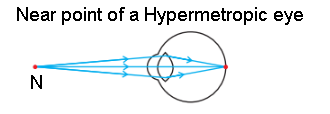Hypermetropia eye:-
f = $\frac{100}{3}$
$P=\frac{1}{f}=\frac{\left(3x100\right)}{\left(100\right)}=3D$

A convex lens of power +3.0 D is required to correct the defect.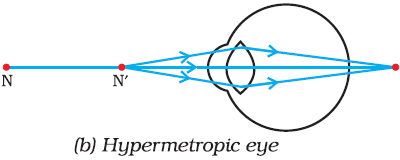Correction of a hypermetropia eye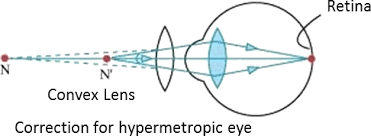Q8 ) Why is a normal eye not able to see clearly the objects placed closer than 25 cm?

NCERT Solutions for Class 10 Science Chapter 11 Human Eye And Colorful World

The image distance in the eye, is fixed and cannot be changed. The ability of the eye lens to adjust its focal length is called accommodation.

Due to this eye can increase or decrease focal length of the lens in order to see either close or distant objects.

In order to see nearby objects the ciliary muscles contract and lens become thick and focal length is decreased which helps to see nearby objects.

In order to see distant objects the ciliary muscles expand and the lens become thin and focal length increases which help to see distant objects.

Q10 ) Why do stars twinkle?

NCERT Solutions for Class 10 Science Chapter 11 Human Eye And Colorful World

Stars twinkle due to atmospheric refraction of starlight. The light from the star on entering earth’s atmosphere undergoes refraction continuously before it reaches the earth.

Refraction occurs and refractive index changes.

As the path of rays of light coming from the star goes on varying slightly, the apparent position of the star fluctuates and the amount of starlight entering the eye flickers.

Therefore sometimes star appears brighter and sometimes twinkle.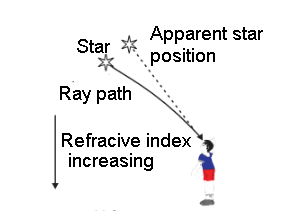Q11 ) Explain why the planets do not twinkle.

NCERT Solutions for Class 10 Science Chapter 11 Human Eye And Colorful World

Planets, do not twinkle as they are closer to the Earth than those distant stars, so planets appear larger in comparison.

Due to the planets’ closeness to Earth, the light coming from them does not bend much due to Earth’s atmosphere.

Therefore, the light coming from our solar system’s planets does not appear to twinkle.

Q12 ) Why does the Sun appear reddish early in the morning?

NCERT Solutions for Class 10 Science Chapter 11 Human Eye And Colorful World

In the early morning, as the Sun rises above horizon, light from the sun has to cover a larger distance in the denser medium of the

earth’s atmosphere before reaching our eyes. The blue light due to shorter wavelength gets scattered in the half way

and not visible in the sky. Only the red light which has larger wavelength is able to reach our eyes due to less scattering.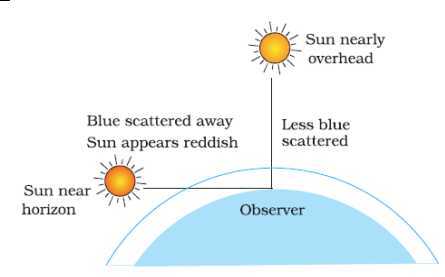Q13 ) Why does the sky appear dark instead of blue to an astronaut?

NCERT Solutions for Class 10 Science Chapter 11 Human Eye And Colorful World

The sky appears dark instead of blue to an astronaut because there is no atmosphere in the outer space that can scatter the sunlight. As the sunlight is not scattered, no scattered light reach the eyes of the astronauts and the sky appears black to them

NCERT solutions for class 10 Science Chapter 11 Human Eye And Colorful World Intext Exercise

Q1 ) What is meant by power of accommodation of the eye ?

NCERT Solutions for Class 10 Science Chapter 11 Human Eye And Colorful World

The power of the eye by which it can see near and far (distant) objects by changing its focal length is called its power of accommodation.

Q2 ) A person with a myopic eye cannot see objects beyond 1.2 m distinctly. What should be the type of the corrective lens used to restore proper vision ?

NCERT Solutions for Class 10 Science Chapter 11 Human Eye And Colorful World

Concave lens

Q3 ) What is the far point and near point of the human eye with normal vision ?

NCERT Solutions for Class 10 Science Chapter 11 Human Eye And Colorful World

For a human eye with normal vision the far point is infinity and the near point is 25 cm.

Q4 ) A student has difficulty reading the blackboard while sitting in the last row. What could be the defect the child is suffering from ? How can it be corrected ?

NCERT Solutions for Class 10 Science Chapter 11 Human Eye And Colorful World

The child is suffering from myopia and the child should use concave lens of suitable focal length.

Q9 ) What happens to the image distance in the eye when we increase the distance of an object from the eye?

NCERT Solutions for Class 10 Science Chapter 11 Human Eye And Colorful World